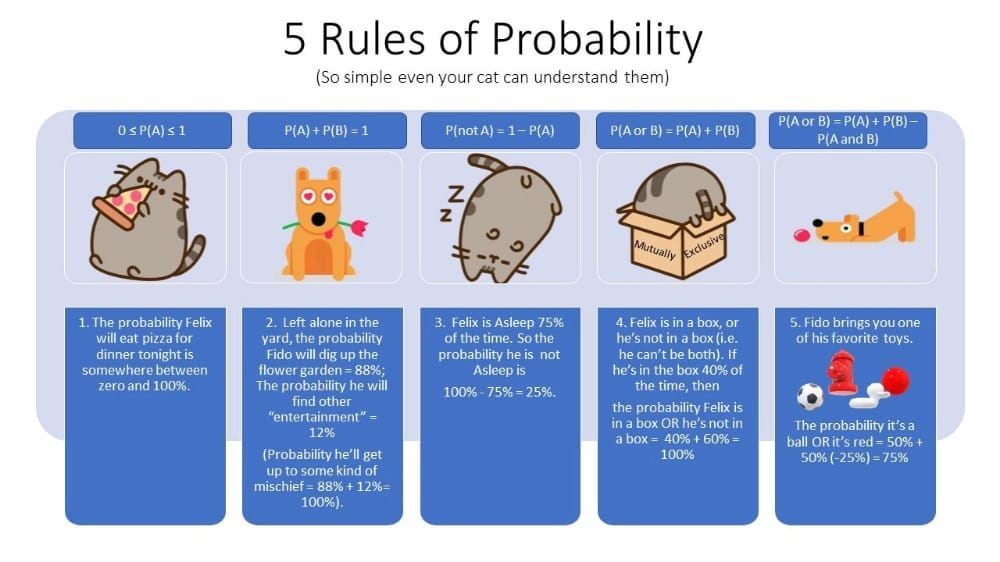# The Math behind Machine Learning# The Math behind Machine Learning

How far is math important when speaking about machine learning?

Many of us start looking at the coding part to improve programming skills.

Machine learning has become a debatable field in the last 5 years, especially after the bomb of ‘Deep Learning’. Within this wide rush towards it, it is important for any beginner in this field to understand the basics of machine learning and its core aspect, the one that is leading it to be here today, and the core that will lead machines to lead the world.

The following image has crossed my way today:

The five rules of probability. Mainly, all programmers have taken a probability course in their high school or college. As simple as it seems, these simple rules form the most important laws of machine learning.

To make things clear, I will start stating some examples that use these probability rules ( shown in the figure above) and others.

CTC algorithm

CTC ( Connectionist Temporal Classification) is one of the interesting algorithms used in sequence-to-sequence models, that is based on probability laws. For instance, it works as follows:

let x be a letter ( x : letter) , w be a word ( w:word)

The question is: what is the probability of having the letter x in the word w ?

p(x/w) = ?. The answer here determines the next step that the algorithm will take.

Now, to know its importance, check the impact of CTC in the following subdomains of machine learning:

Handwritten text recognition, Voice recognition

The reason behind using ctc in these models is that they rely mainly on the probability on the next step to decide the current one.

The figure above shows the baseline architecture of the handwritten decoding model. First of all, convolutional layers are used to extract features for the handwritten images. Next, their output is fed to bidirectional lstm layers, simply to save the current feature(letter) to compare it with the next feature(letter). In this case, we say what is the probability of letter x1 knowing that it is followed ( or passed by) letter x2? see? simple probability lows lead to gentle and great models that will take the world into another future!

Finally, after predicting the pattern of letters, they will enter into a decision-making tree, the CTC model is responsible for choosing the correct pattern of the word.

Probability is the science of studying uncertainty. Machine learning is the science of solving uncertainty by making it ‘mostly’ certain.

These two factors make a great combination of togerther.

In conclusion, never underestimate the power of math. It can make miracles.